Tamilnadu State Board New Syllabus Samacheer Kalvi 11th Maths Guide Pdf Chapter 10 Differentiability and Methods of Differentiation Ex 10.5 Text Book Back Questions and Answers, Notes.

## Tamilnadu Samacheer Kalvi 11th Maths Solutions Chapter 10 Differentiability and Methods of Differentiation Ex 10.5

Choose the correct or the most suitable answer from the given four alternatives.

Question 1.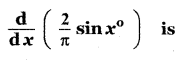(1) $$\frac{\pi}{180}$$ cos x°
(2) $$\frac{1}{90}$$ cos x°
(3) $$\frac{\pi}{90}$$ cos x°
(4) $$\frac{2}{\pi}$$ cos x°
(2) $$\frac{1}{90}$$ cos x°

Explaination: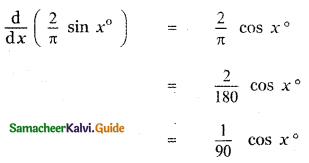Question 2.
If y = f(x2 + 2) and f'(3) = 5 , then $$\frac{\mathrm{dy}}{\mathrm{d} x}$$ at x = 1 is
(1) 5
(2) 25
(3) 15
(4) 10
(4) 10

Explaination:
y = f(x2 + 2)
$$\frac{\mathrm{dy}}{\mathrm{d} x}$$ = f’ (x2 + 2) × 2x
$$\frac{\mathrm{dy}}{\mathrm{d} x} / x=1$$ = f’ (12 + 2) × 2 × 1
= f’(3) × 2
= 5 × 2 = 10

Question 3.
If y = $$\frac{1}{4}$$u4, u = $$\frac{2}{3}$$ x3 + 5, then $$\frac{\mathrm{dy}}{\mathrm{d} x}$$ is
(1) $$\frac{1}{27}$$ x2 (2x3 + 15)3
(2) $$\frac{2}{27}=$$ x (2x3 + 5)3
(3) $$\frac{2}{27}=$$ x2 (2x3 + 15)3
(4) – $$\frac{2}{27}=$$ x (2x3 + 5)3
(3) $$\frac{2}{27}=$$ x2 (2x3 + 15)3

Explaination: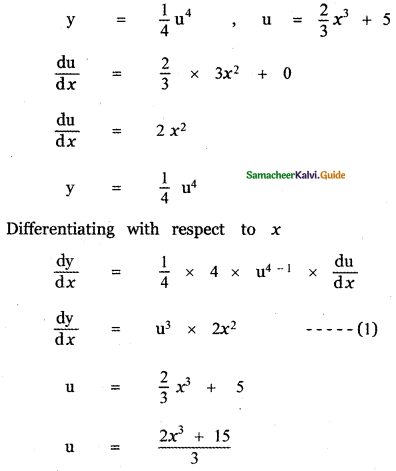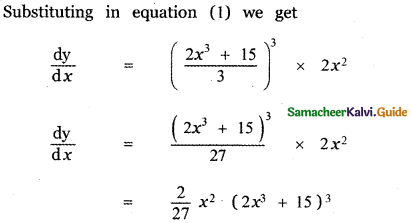Question 4.
If f(x) = x2 – 3x, then the points at which f (x) = f’ (x) are
(1) both positive integers
(2) both negative integers
(3) both irrational
(4) one rational and another irrational
(3) both irrational

Explaination:
f(x) = x2 – 3x
f’(x) = 2x – 3
f(x) = f'(x)
⇒ x2 – 3x = 2x – 3
x2 – 3x – 2x + 3 = 0
x2 – 5x + 3 = 0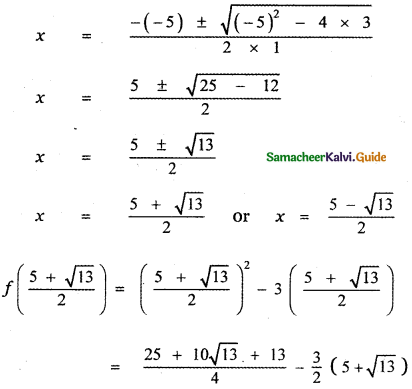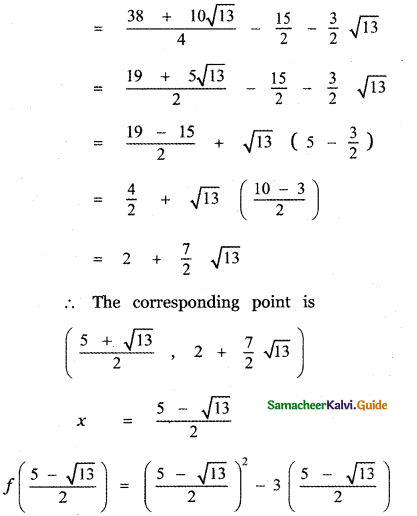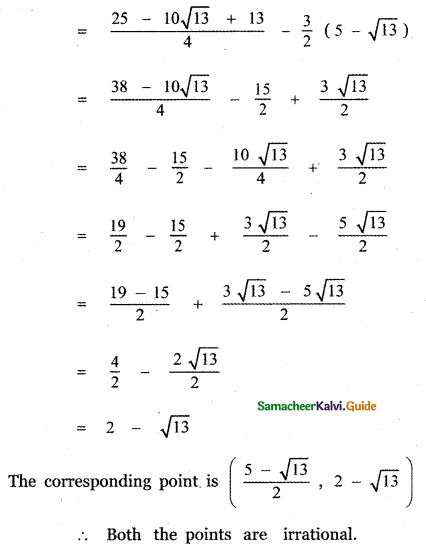Question 5.
If y = $$\frac{1}{a-z}$$, then $$\frac{\mathrm{d} \mathrm{z}}{\mathrm{d} \mathrm{y}}$$ is
(1) (a – z)2
(2) – (z – a)2
(3) (z + a)2
(4) – (z + a)2
(1) (a – z)2

Explaination: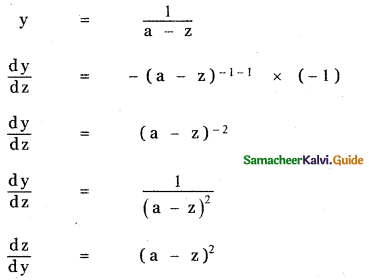Question 6.
If y = cos (sin x2), then $$\frac{\mathrm{dy}}{\mathrm{d} x}$$ at x = $$\sqrt{\frac{\pi}{2}}$$ is
(1) – 2
(2) 2
(3) – 2 $$\sqrt{\frac{\pi}{2}}$$
(4) 0
(4) 0

Explaination: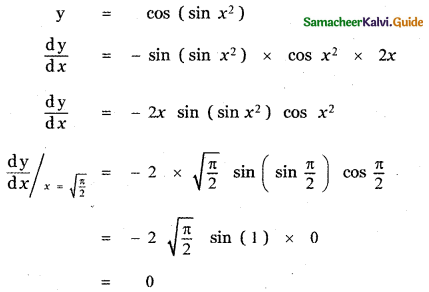Question 7.
If y = mx + c and f(0) = f’(0) = 1, then f(2) is
(1) 1
(2) 2
(3) 3
(4) – 3
(3) 3

Explaination:
y = mx+c
$$\frac{d y}{d x}$$ = m
y = x + c (i.e.) f(x) = x + c
y(a tx = 0) = f(0) 0 + c = 1 ⇒ c = 1
y = x + 1 ⇒ f(x) = x + 1
f(2) = 2 + 1 = 3

Question 8.
If f(x) = x tan-1 x then f'(x) is
(1) $$1+\frac{\pi}{4}$$
(2) $$\frac{1}{2}+\frac{\pi}{4}$$
(3) $$\frac{1}{2}-\frac{\pi}{4}$$
(4) 2
(2) $$\frac{1}{2}+\frac{\pi}{4}$$

Explaination: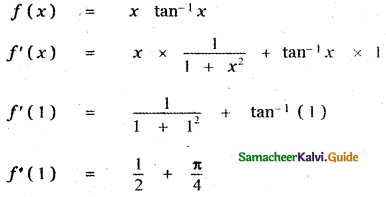Question 9.
$$\frac{d}{d x}$$ (ex + 5 log x) is
(1) ex . x4 (x + 5)
(2) ex . x (x + 5)
(3) ex + $$\frac{5}{x}$$
(4) ex – $$\frac{5}{x}$$
(1) ex . x4 (x + 5)

Explaination: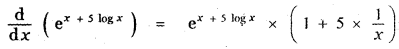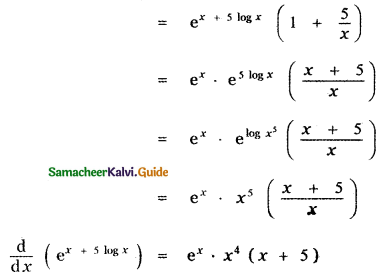Question 10.
If the derivative of (ax – 5)e at x = 0 is – 13 , then the value of a ¡s
(1) 8
(2) – 2
(3) 5
(4) 2
(4) 2

Explaination:
y = (ax – 5)e3x
$$\frac{d y}{d x}$$ = y’ = (ax – 5) (3e3x) + e3x (a)
= e3x[3ax – 15 + a]
Given $$\frac{d y}{d x}$$ = -13 at x = 0
⇒ [-15 + a] = -13
⇒ a = -13 + 15
a = 2Question 11.
x = $$\frac{1-t^{2}}{1+t^{2}}$$, y = $$\frac{2 t}{1+t^{2}}$$ then $$\frac{d y}{d x}$$ is
(1) – $$\frac{\mathbf{y}}{x}$$
(2) $$\frac{\mathbf{y}}{x}$$
(3) – $$\frac{x}{y}$$
(4) $$\frac{x}{y}$$
(3) – $$\frac{x}{y}$$

Explaination: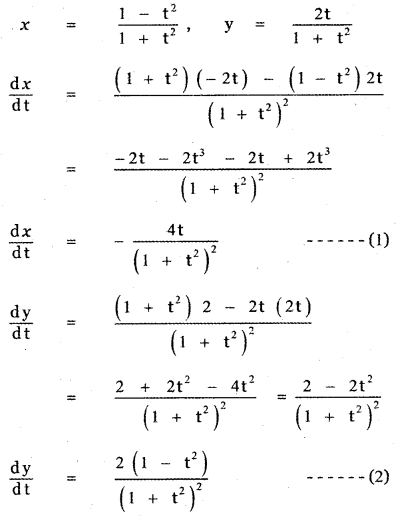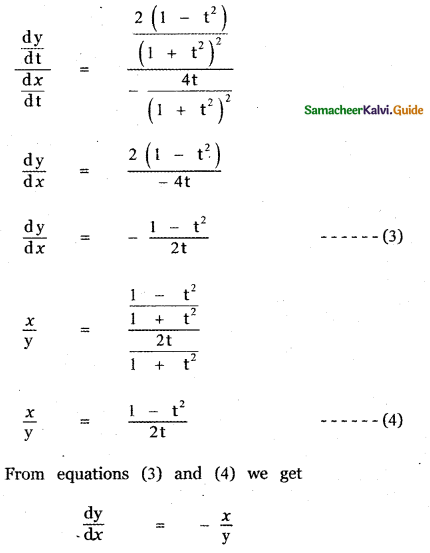Question 12.
If x = a sin θ and y = b cos θ, then  is
(1) $$\frac{\mathbf{a}}{\mathbf{b}^{2}}$$ sec2 θ
(2) $$-\frac{\mathbf{b}}{\mathbf{a}}$$ sec2 θ
(3) $$-\frac{b}{a^{2}}$$ sec3 θ
(4) $$-\frac{b^{2}}{a^{2}}$$ sec3 θ
(3) $$-\frac{b}{a^{2}}$$ sec3 θ

Explaination: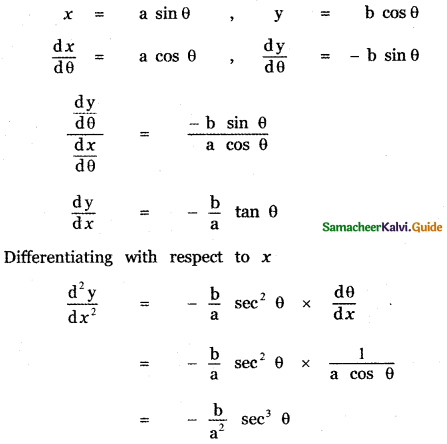Question 13.
The differential coefficient of log10 x with respect to log10 x is
(1) 1
(2) – (log10 x)2
(3) (log10 x)2
(4) $$\frac{x^{2}}{100}$$
(2) – (log10 x)2

Explaination: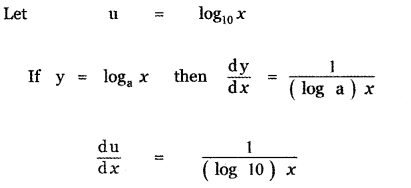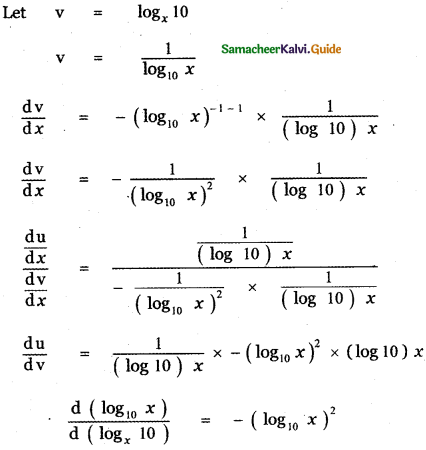Question 14.
If f(x) = x + 2 , then f’ (f(x)) at x= 4 is
(1) 8
(2) 1
(3) 4
(4) 5
(2) 1

Explaination:
f(x) x + 2
f’ (f(x)) = $$\frac{\mathrm{d}}{\mathrm{d} x}$$ (f(x))
= $$\frac{\mathrm{d}}{\mathrm{d} x}$$ (x + 2) = 1

Question 15.
If y = $$\frac{(1-x)^{2}}{x^{2}}$$, then $$\frac{\mathrm{dy}}{\mathrm{d} x}$$ is
(1) $$\frac{2}{x^{2}}+\frac{2}{x^{3}}$$
(2) $$-\frac{2}{x^{2}}+\frac{2}{x^{3}}$$
(3) $$-\frac{2}{x^{2}}-\frac{2}{x^{3}}$$
(4) $$-\frac{2}{x^{3}}+\frac{2}{x^{2}}$$
(4) y = $$\frac{(1-x)^{2}}{x^{2}}$$

Explaination: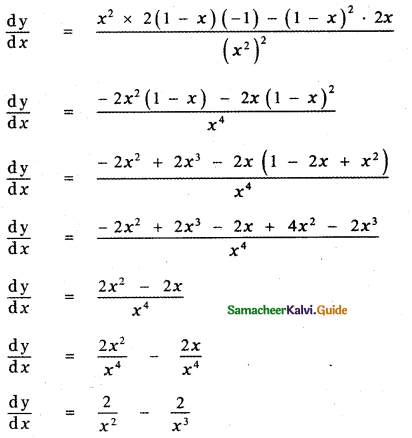Question 16.
If pv = 81, then $$\frac{\mathbf{d} \mathbf{p}}{\mathbf{d v}}$$ at v = 9 is
(1) 1
(2) – 1
(3) 2
(4) – 3
(2) – 1

Explaination: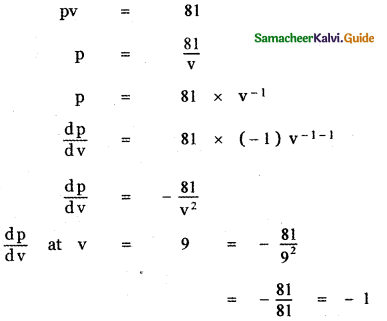Question 17.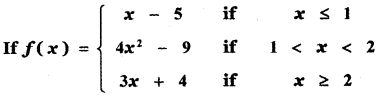(1) 0
(2) 2
(3) 3
(4) 4
(3) 3

Explaination: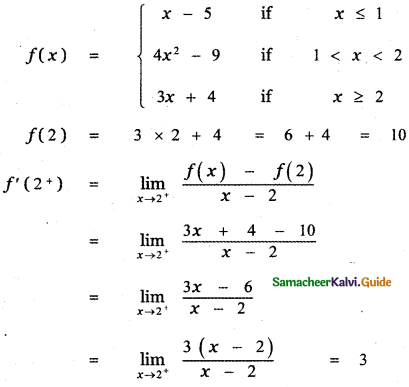Question 18.
It is given that f’ (a) exists, then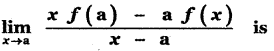(1) f(a) – af'(a)
(2) f'(a)
(3) – f'(a)
(4) f(a) + af'(a)
(1) f(a) – af'(a)

Explaination: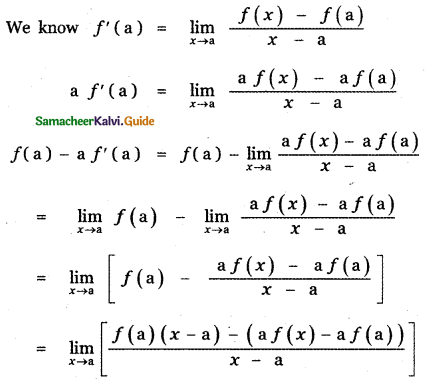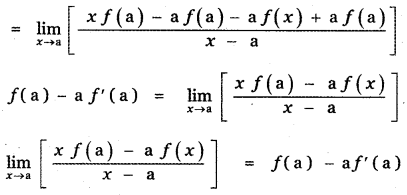Question 19.
If f(x) =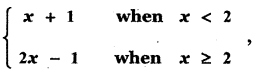then f’ (2) is
(1) 0
(2) 1
(3) 2
(4) does not exist
(3) 2

Explaination: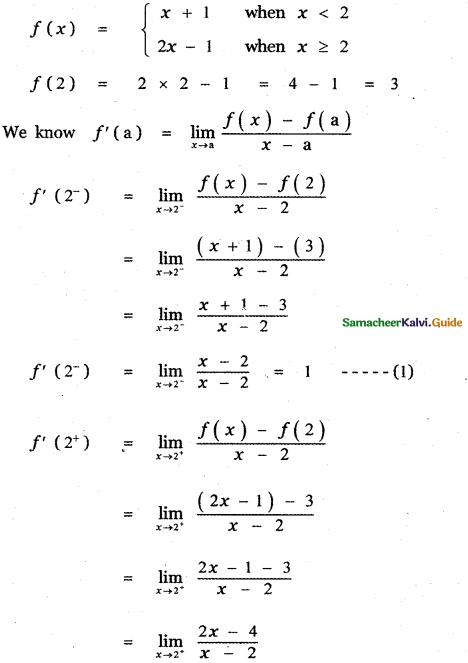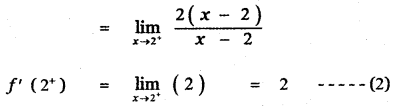Question 20.
If g(x) = (x2 + 2x + 3) f(x) and f(0) – 5 and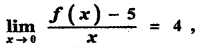then g'(0) is
(1) 20
(2) 14
(3) 18
(4) 12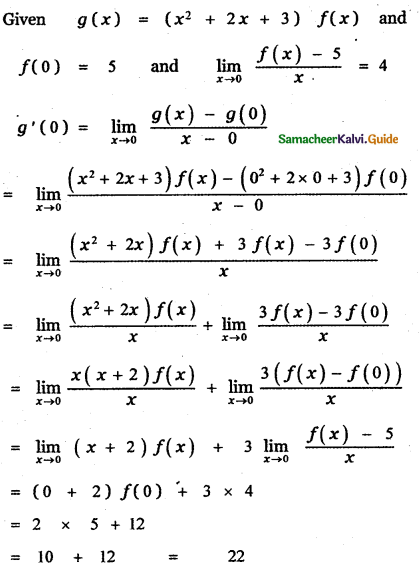Question 21.
If f(x) =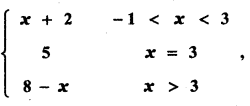then at x = 3, f'(x) is
(1) 1
(2) – 1
(3) 0
(4) does not exist
(2) – 1

Explaination: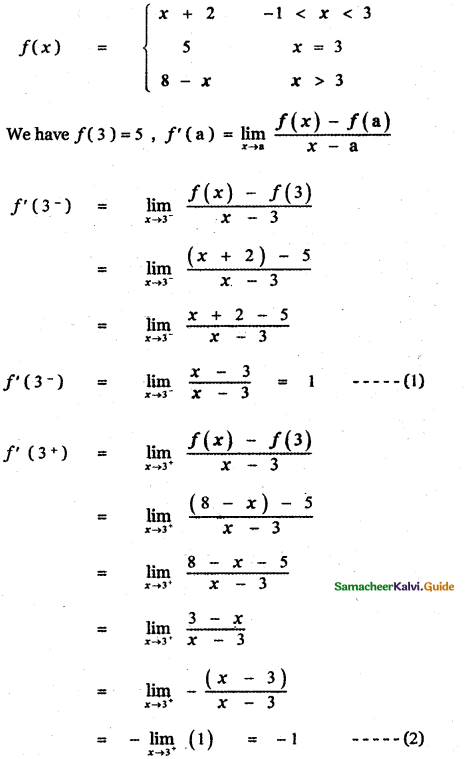From equations (1) and (2) we get
f’ (3) ≠ f’ (3+)
∴ limit of f(x) does not exist at x = 3
f’ (x) does not exist at x = 3

Question 22.
The derivative of f(x)= x|x| at x = – 3 is
(1) 6
(2) – 6
(3) does not exist
(4) 0
(1) 6

Explaination:
f(x) = x|x|
f(x) = x(-x) ⇒ f(x) = – x2
f ‘(x) = -(2x)
f ‘(-3) = -(2) (-3) = 6Question 23.
If f(x) =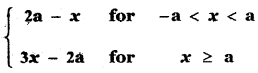then which one of the following is true?
(1) f(x) is not differentiable at x = a
(2) f(x) is discontinuous at x = a
(3) f(x) is continuous for all x in R
(4) f(x) is differentiable for all x ≥ a
(1) f(x) is not differentiable at x = a

Explaination: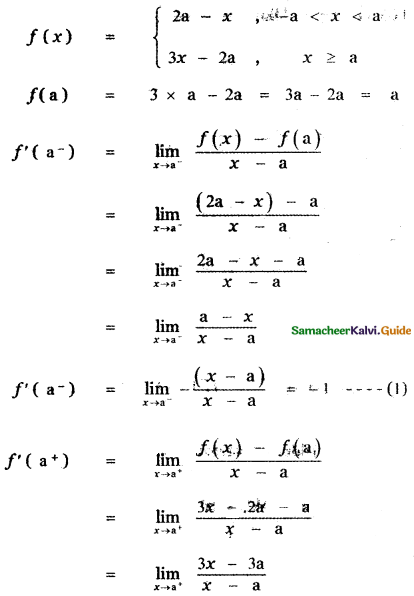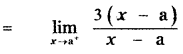f’ (a+) = 3 ………. (2)
From equations (1) and (2) we get
f'(a) ≠ f'(a+)
∴ f’ (x) does not exist at x = a
∴ f(x) is not differentiable at x = aQuestion 24.
If f(x) =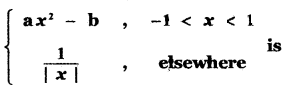is differentiable at x = 1, then
(1) a = $$\frac{1}{2}$$, b = $$\frac{-3}{2}$$
(2) a = $$\frac{-1}{2}$$, b = $$\frac{3}{2}$$
(3) a = $$-\frac{1}{2}$$, b = $$-\frac{3}{2}$$
(4) a = $$\frac{1}{2}$$, b = $$\frac{3}{2}$$
(3) a = $$-\frac{1}{2}$$, b = $$-\frac{3}{2}$$

Explaination: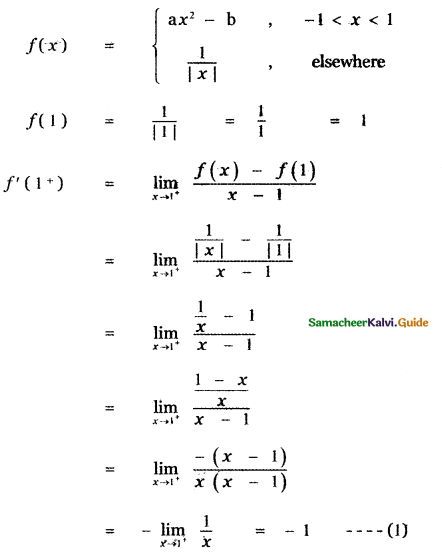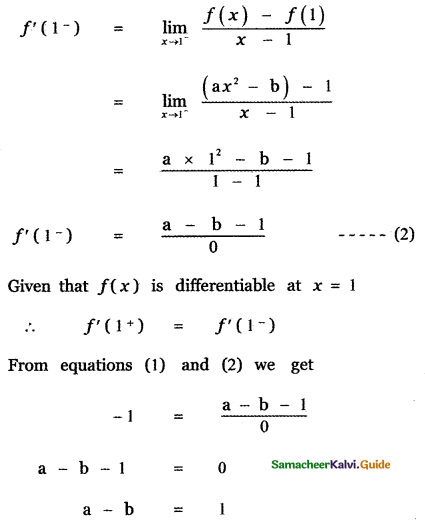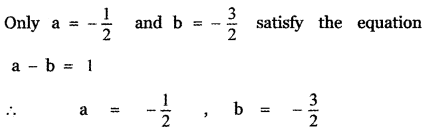Question 25.
The number of points in R in which the function f(x) = |x – 1| + |x – 3| + sin x is not differentiable, is
(1) 3
(2) 2
(3) 1
(4) 4